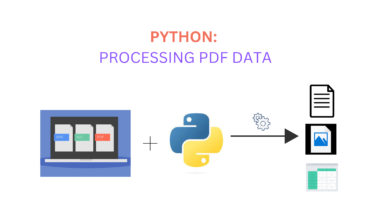# How to read data from csv using python

CSV files are a common format for storing tabular data, making them ideal for tasks such as data analysis and machine learning. Python, with its robust libraries, offers several methods to read data from CSV files easily. In this blog post, we’ll explore methods to read data from CSV files using Python, providing examples and best practices along the way.

## Introduction to CSV Files

CSV (Comma Separated Values) is a simple file format that uses commas to separate values. Each line in a CSV file corresponds to a row in the table, with each value in that line corresponding to a column.

Example of a CSV file:

``````Name, Age, City
John Doe, 28, New York
Jane Smith, 34, Los Angeles``````

Python has multiple methods to read CSV files, but the two most common ones include:

1. Using Python’s Built-in csv Module
2. Using the pandas Library

## Method 1: Using Python’s Built-in csv Module

The csv module in Python provides functionality to work with CSV files directly.

Step-by-Step Guide:

1. Import the csv module

``import csv``

2. Open the CSV file

``````with open('example.csv', 'r') as file:

``header = next(csvreader)``

4. Iterating through rows

``````for row in csvreader:
print(row)``````

5. Complete example

``````import csv
with open('example.csv', 'r') as file:
print(row)``````

## Method 2: Using the pandas Library

pandas is a powerful library for data analysis and manipulation. It can read CSV files directly into a DataFrame, an in-memory 2D data structure similar to a table.

Step-by-Step Guide:

1. Install pandas (if not already installed)

``pip install pandas``

2. Import pandas

``import pandas as pd``

``df = pd.read_csv('example.csv')``

4. Accessing data

``print(df.head())``

5. Complete example:

``````import pandas as pd

print(df)``````

## When to Use csv Module vs. pandas

• Use the csv module when dealing with simple CSV files where pandas might be an overkill.
• Use pandas for more complex actions like data cleaning, analysis, and visualization.

## Conclusion

Reading CSV files in Python is straightforward with the built-in csv module for simple tasks or the pandas library for robust data manipulation. Understanding these methods increases effectiveness when dealing with data in your Python applications.